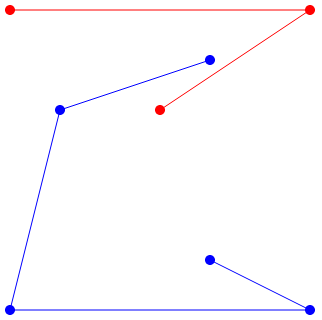CSES - HIIT Open 2019 - Keeping Distance
• Time limit: 1.00 s
• Memory limit: 512 MB

You are given a set of n distinct points in the plane. Each point has integer coordinates between 0 \dots k, and is either blue or red.

There are always four points with fixed coordinates: (0,0) and (k,0), which are blue, and (0,k) and (k,k), which are red. The other points may have any coordinates and colors, but there are never three points on the same line.

Your task is to add n-2 line segments, each of which connects two points of the same color, in such a way that all blue and red points belong to the same component, and no two line segments intersect.

# Input

The first input line has two integers n and k: the number of points and the coordinate upper bound.

After this, there are n lines that describe the points. Each line has three integers x, y and c: a point is located at (x,y) and its color is blue (c=1) or red (c=2).

# Output

Print n-2 lines that describe the connections. Each line must have four integers x_1, y_1, x_2 and y_2: the points (x_1,y_1) and (x_2,y_2) are connected.

You can print any valid solution, and it can be proven that there is always a solution.

# Constraints

• 4 \le n \le 1000
• 1 \le k \le 10^9

# Example

Input:

8 6
0 0 1
0 6 2
1 4 1
3 4 2
4 1 1
4 5 1
6 0 1
6 6 2


Output:

0 0 1 4
0 0 6 0
0 6 6 6
1 4 4 5
6 0 4 1
6 6 3 4


The following figure corresponds to this example: